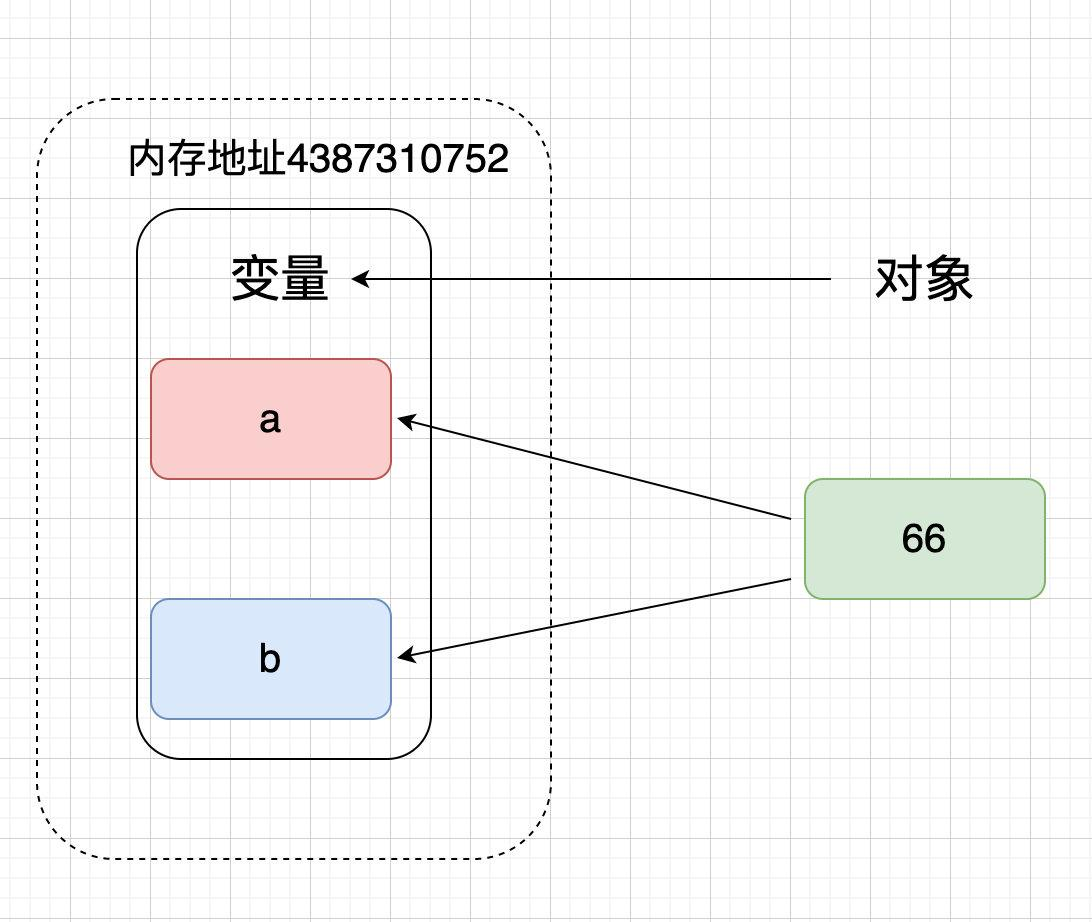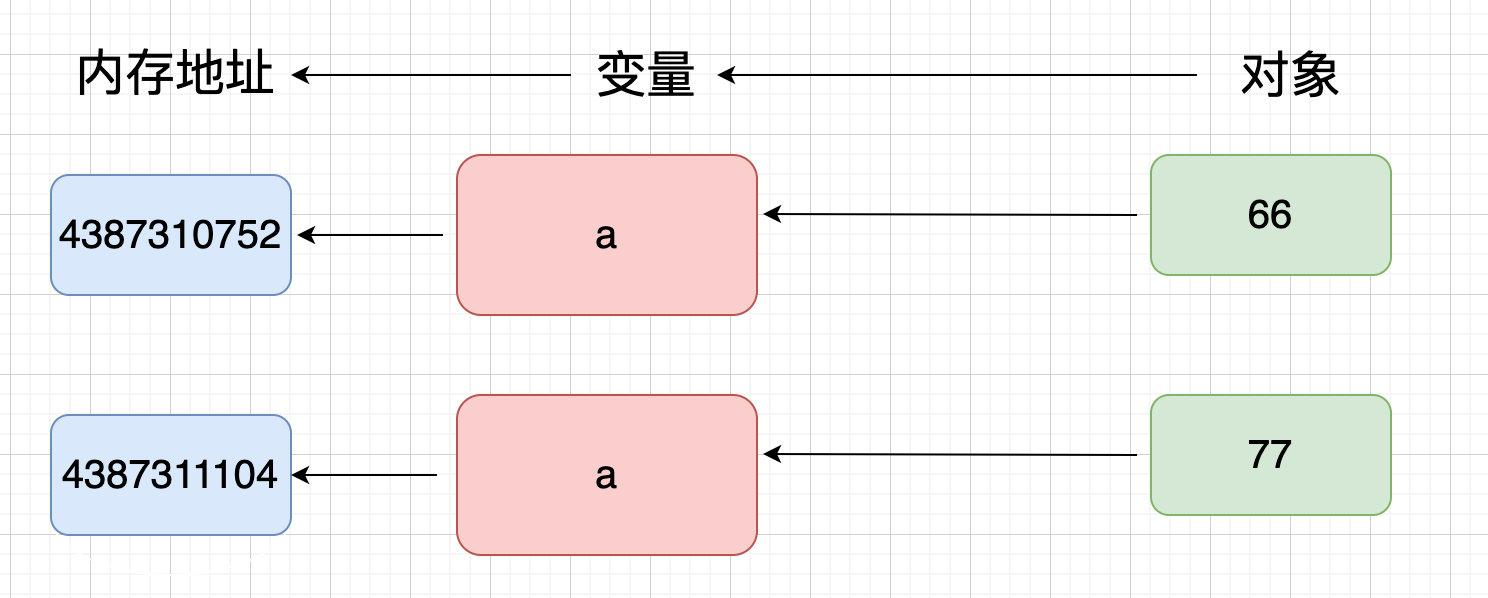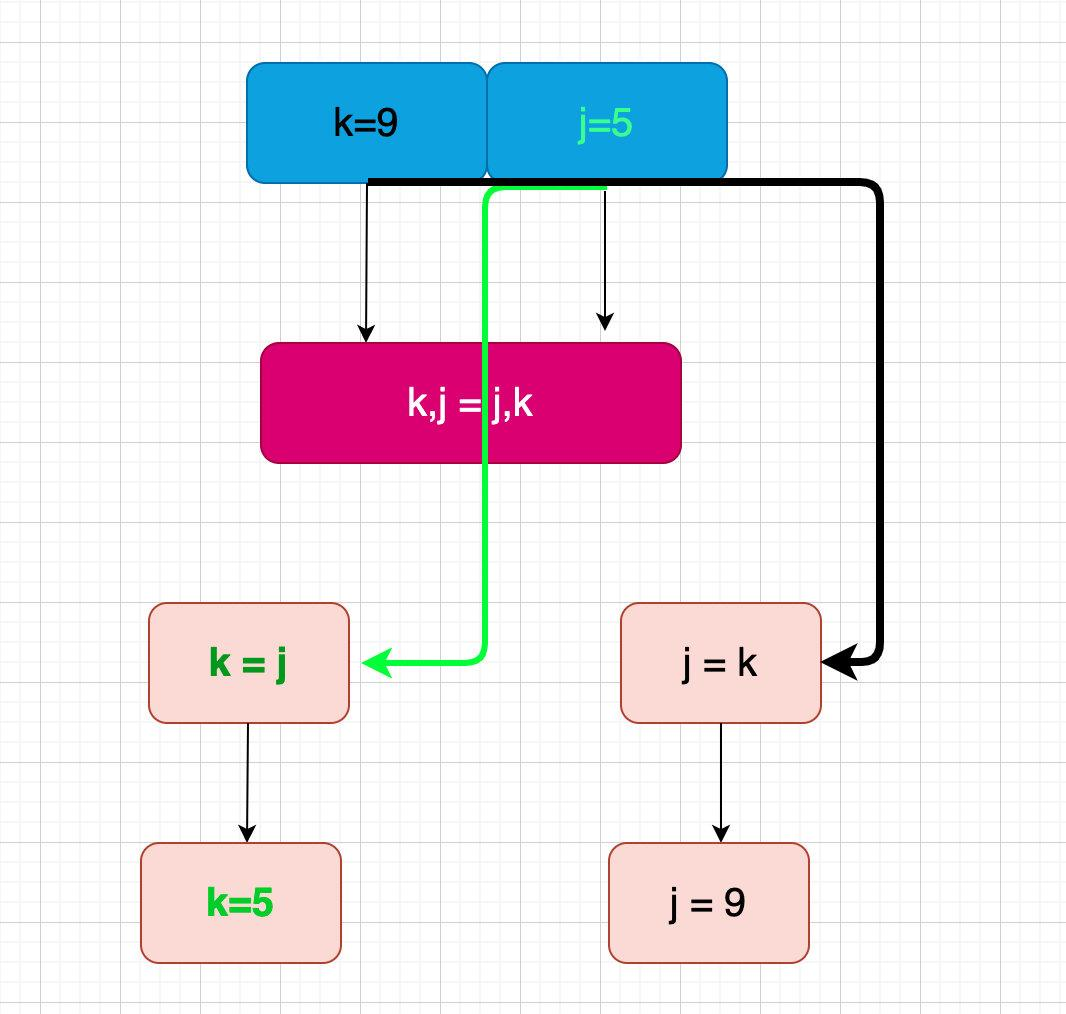# Python入門：Python變數和賦值

## theme: smartblue## 環境

• 系統：MacOS
• 工具：jupyter notebook
• Python版本：3.7.5
• 文件編輯器：Typora

## 變數

### 深刻理解變數的記憶體地址

```python a = 66 a```

``````66
``````

```python b = 66 b```

``````66
``````

```python type(a) # 檢視數值型別為整型int```

``````int
``````

```python type(b) # 字串型別```

``````int
``````

```python id(a) # a和b的記憶體地址相同```

``````4387310752
``````

```python id(b)```

``````4387310752
`````````python a = 77 a```

``````77
``````

```python id(a)```

``````4387311104
``````### 建立變數

```python x = 99 # 數值型 language = "python" # 字串型 number = [1,3,5,7,9] # 列表型

print(x) print(language) print(number) ```

``````99
python
[1, 3, 5, 7, 9]
``````

### 變數名稱命名規則

python中變數的命名可以使用短名稱，比如上面的x、y、z、a、b等，也可以使用具有一定描述作用的名稱，比如age、name、sex，其他人看到就可以知道變數的含義。通常Python中的變數命名規則：

• 變數名必須以字母或下劃線字元開頭，不能以數字開頭
• 變數名只能包含字母數字字元和下劃線（A-z、0-9 和 _）
• 變數名稱區分大小寫（name、Name 和 NAME 就是三個不同的變數）
• 變數名不能和Python中的關鍵字衝突（相同），否則無效

## 賦值語句

### 常規賦值

```python name = value

# 變數 = 某個值

```

```python list1 = ["python","java"] # 列表賦值給變數b list1```

``````['python', 'java']
``````

```python list2 = [1,2,["python","html"],(1,4,7)] # 巢狀列表 list2```

``````[1, 2, ['python', 'html'], (1, 4, 7)]
``````

```python age = 28 # 數值 age```

``````28
``````

```python information = "xiaoming is a boy" # 字串 information```

``````'xiaoming is a boy'
``````

```python

# 定義一個變數dic，字典型別

dic = {"name":"xiaoming","age":20,"sex":"fale"} dic ```

``````{'name': 'xiaoming', 'age': 20, 'sex': 'fale'}
``````

### 多變數賦值

```python m, n, o= 22, "xiaoming","男" # 同時賦值3個變數```

```python m```

``````22
``````

```python n```

``````'xiaoming'
``````

```python o```

``````'男'
``````

```python name, age = ("Peter",20) # 通過元組形式賦值```

```python name```

``````'Peter'
``````

```python age```

``````20
``````

### 鏈式賦值

```python x1 = y1 = 33```

```python x1```

``````33
``````

```python y1```

``````33
``````

```python id(x1)```

``````4387309696
``````

```python id(y1)```

``````4387309696
``````

### 變數互換

```python k, j = 9, 5```

```python k=9 j=5```

```python print("k =",k) print("j =",j)```

``````k = 9
j = 5
``````

```python print("id(k): ", id(k)) print("id(j): ", id(j))```

``````id(k):  4387308928
id(j):  4387308800
``````

```python k, j = j, k # 變數值的交換```

```python print("k =",k) print("j =",j)```

``````k = 5
j = 9
`````````python print("id(k): ", id(k)) print("id(j): ", id(j))```

``````id(k):  4387308800
id(j):  4387308928
``````

```python temp = a # a賦值中間變數temp a = b # b的值賦給變數a b = temp # temp的值賦給變數b```

## 變數的相等和相同

```python number1 = 88 number2 = 88```

```python id(number1)```

``````4387311456
``````

```python id(number2)```

``````4387311456
``````

```python number1 == number2```

``````True
``````

```python number1 is number2```

``````True
``````

```python list1 = "hello python" list2 = "hello python"```

```python list1 == list2 # 相等```

``````True
``````

```python list1 is list2 # 不相同```

``````False
``````

```python id(list1)```

``````4444494000
``````

```python id(list2)```

``````4444495024
``````

```python number3 = 1000 number4 = 1000```

```python number3 == number4 # 相等```

``````True
``````

```python number3 is number4 # 居然不相等啦！```

``````False
``````

```python id(number3)```

``````4444408880
``````

```python id(number4)```

``````4444409104
``````

「其他文章」